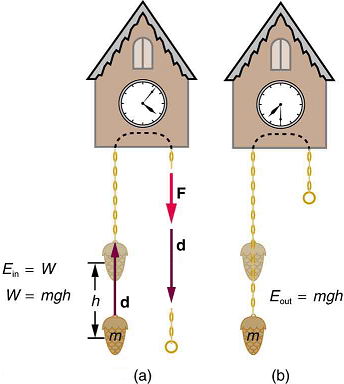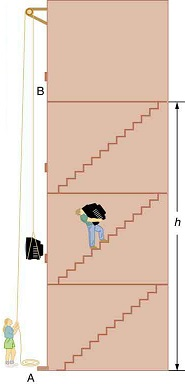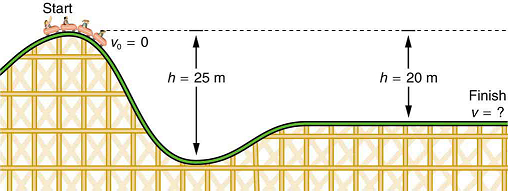$$\require{cancel}$$

# 7.4: Gravitational Potential Energy

•• Contributed by OpenStax
• General Physics at OpenStax CNX

# Work Done Against Gravity

Climbing stairs and lifting objects is work in both the scientific and everyday sense—it is work done against the gravitational force. When there is work, there is a transformation of energy. The work done against the gravitational force goes into an important form of stored energy that we will explore in this section.

Let us calculate the work done in lifting an object of mass $$m$$ through a height $$h$$ such as in Figure. If the object is lifted straight up at constant speed, then the force needed to lift it is equal to its weight $$mg$$. The work done on the mass is then $$W = Fd = mgh$$. We define this to be the gravitational potential energy $$(PE_g)$$ put into (or gained by) the object-Earth system. This energy is associated with the state of separation between two objects that attract each other by the gravitational force. For convenience, we refer to this as the $$PE_g$$ gained by the object, recognizing that this is energy stored in the gravitational field of Earth. Why do we use the word “system”? Potential energy is a property of a system rather than of a single object—due to its physical position. An object’s gravitational potential is due to its position relative to the surroundings within the Earth-object system. The force applied to the object is an external force, from outside the system. When it does positive work it increases the gravitational potential energy of the system. Because gravitational potential energy depends on relative position, we need a reference level at which to set the potential energy equal to 0. We usually choose this point to be Earth’s surface, but this point is arbitrary; what is important is the difference in gravitational potential energy, because this difference is what relates to the work done. The difference in gravitational potential energy of an object (in the Earth-object system) between two rungs of a ladder will be the same for the first two rungs as for the last two rungs.

# Converting Between Potential Energy and Kinetic Energy

Gravitational potential energy may be converted to other forms of energy, such as kinetic energy. If we release the mass, gravitational force will do an amount of work equal to $$mgh$$ on it, thereby increasing its kinetic energy by that same amount (by the work-energy theorem). We will find it more useful to consider just the conversion of $$PE_g$$ to $$KE$$ without explicitly considering the intermediate step of work. (See Example $$\PageIndex{2}$$.) This shortcut makes it is easier to solve problems using energy (if possible) rather than explicitly using forces.Figure $$\PageIndex{1}$$: (a) The work done to lift the weight is stored in the mass-Earth system as gravitational potential energy. (b) As the weight moves downward, this gravitational potential energy is transferred to the cuckoo clock.

More precisely, we define the change in gravitational potential energy $$\Delta PE_g$$ to be

$\Delta PE_g = mgh,$

where, for simplicity, we denote the change in height by $$h$$ rather than the usual $$\Delta h$$. Note that $$h$$ is positive when the final height is greater than the initial height, and vice versa. For example, if a 0.500-kg mass hung from a cuckoo clock is raised 1.00 m, then its change in gravitational potential energy is

$mgh = (0.500 \, kg)(9.80 \, m/s^2)(1.00 \, m)$

$= 4.90 \, kg \cdot m^2/s^2 = 4.90 \, J.$

Note that the units of gravitational potential energy turn out to be joules, the same as for work and other forms of energy. As the clock runs, the mass is lowered. We can think of the mass as gradually giving up its 4.90 J of gravitational potential energy, without directly considering the force of gravity that does the work.

# Using Potential Energy to Simplify Calculations

The equation $$\Delta PE_g = mgh$$ applies for any path that has a change in height of $$h$$, not just when the mass is lifted straight up. (See Figure.) It is much easier to calculate $$mgh$$ (a simple multiplication) than it is to calculate the work done along a complicated path. The idea of gravitational potential energy has the double advantage that it is very broadly applicable and it makes calculations easier. From now on, we will consider that any change in vertical position $$h$$ of a mass $$m$$ is accompanied by a change in gravitational potential energy $$mgh$$, and we will avoid the equivalent but more difficult task of calculating work done by or against the gravitational force.Figure $$\PageIndex{2}$$: The change in gravitational potential energy $$(\Delta PE_g)$$ between points A and B is independent of the path $$\Delta PE_g = mgh$$ for any path between the two points. Gravity is one of a small class of forces where the work done by or against the force depends only on the starting and ending points, not on the path between them.

Example $$\PageIndex{1}$$: The Force to Stop Falling

A 60.0-kg person jumps onto the floor from a height of 3.00 m. If he lands stiffly (with his knee joints compressing by 0.500 cm), calculate the force on the knee joints.

Strategy

This person’s energy is brought to zero in this situation by the work done on him by the floor as he stops. The initial $$PE_g$$ is transformed into $$KE$$ as he falls. The work done by the floor reduces this kinetic energy to zero.

Solution

The work done on the person by the floor as he stops is given by

$W = Fd \, cos \, \theta = - Fd$

with a minus sign because the displacement while stopping and the force from floor are in opposite directions $$(cos \, \theta = cos \, 180^o = -1.)$$ The floor removes energy from the system, so it does negative work.

The kinetic energy the person has upon reaching the floor is the amount of potential energy lost by falling through height $$h$$:

$KE = -\Delta PE_g = -mgh$

The distance $$d$$ that the person’s knees bend is much smaller than the height $$h$$ of the fall, so the additional change in gravitational potential energy during the knee bend is ignored. The work $$W$$ done by the floor on the person stops the person and brings the person’s kinetic energy to zero:

$W = -KE = mgh$

Combining this equation with the expression for $$W$$ gives $-Fd = mgh.$

Recalling that $$h$$ is negative because the person fell down, the force on the knee joints is given by

$F = -\dfrac{mgh}{d} = -\dfrac{(60.0 \, kg)(9.80 \, m/s^2)(-3.00 \, m)}{5.00 \times 10^{-3} \, m} = 3.53 \times 10^5 \, N.$

Discussion

Such a large force (500 times more than the person’s weight) over the short impact time is enough to break bones. A much better way to cushion the shock is by bending the legs or rolling on the ground, increasing the time over which the force acts. A bending motion of 0.5 m this way yields a force 100 times smaller than in the example. A kangaroo's hopping shows this method in action. The kangaroo is the only large animal to use hopping for locomotion, but the shock in hopping is cushioned by the bending of its hind legs in each jump.(See Figure.)Figure $$\PageIndex{3}$$: The work done by the ground upon the kangaroo reduces its kinetic energy to zero as it lands. However, by applying the force of the ground on the hind legs over a longer distance, the impact on the bones is reduced. (credit: Chris Samuel, Flickr)

Example $$\PageIndex{2}$$: Finding the Speed of a Roller Coaster from its Height

(a) What is the final speed of the roller coaster shown in Figure, if it starts from rest at the top of the 20.0 m hill and work done by frictional forces is negligible? (b) What is its final speed (again assuming negligible friction) if its initial speed is 5.00 m/s?Figure $$\PageIndex{4}$$: The speed of a roller coaster increases as gravity pulls it downhill and is greatest at its lowest point. Viewed in terms of energy, the roller-coaster-Earth system’s gravitational potential energy is converted to kinetic energy. If work done by friction is negligible, all $$\Delta PE_g$$ is converted to $$KE$$.

Strategy

The roller coaster loses potential energy as it goes downhill. We neglect friction, so that the remaining force exerted by the track is the normal force, which is perpendicular to the direction of motion and does no work. The net work on the roller coaster is then done by gravity alone. The loss of gravitational potential energy from moving downward through a distance $$h$$ equals the gain in kinetic energy. This can be written in equation form as $$-\Delta PE = \Delta KE$$. Using the equations for $$PE_g$$ and $$KE$$ we can solve for the final speed $$v$$, which is the desired quantity.

Solution for (a)

Here the initial kinetic energy is zero, so that $$\Delta KE = \frac{1}{2}mv^2$$. The equation for change in potential energy states that $$\Delta PE_g = mgh$$. Since $$h$$ is negative in this case, we will rewrite this as $$\Delta PE_g = -mg|h|$$ to show the minus sign clearly. Thus,$-\Delta PE_g = \Delta KE$ becomes $mg|h| = \dfrac{1}{2}mv^2$

Solving for $$v$$ we find that mass cancels and that $v = \sqrt{2g|h|}.$

Substituting known values,

$v = \sqrt{2(9.80 \, m/s^2)(20.0 \, m)}$

$= 19.8 \, m/s$

Solution for (b)

Again $$-\Delta PE_g = \Delta KE$$. In this case there is initial kinetic energy, so $$\Delta KE = \frac{1}{2}mv^2 - \frac{1}{2}mv_0^2$$. Thus,

$mgh = \dfrac{1}{2}mv^2 - \dfrac{1}{2}m_0^2.$

Rearranging gives

$\dfrac{1}{2}mv^2 = mg|h| + \dfrac{1}{2}mv_0^2.$

This means that the final kinetic energy is the sum of the initial kinetic energy and the gravitational potential energy. Mass again cancels, and $v = \sqrt{2g|h| + v_0^2}.$

This equation is very similar to the kinematics equation $$v = \sqrt{v_0^2 + 2ad}$$, but it is more general—the kinematics equation is valid only for constant acceleration, whereas our equation above is valid for any path regardless of whether the object moves with a constant acceleration. Now, substituting known values gives

$v = \sqrt{2(9.80 \, m/s^2)(20.0 \, m) + (5.00)^2}$

$= 20.4 \, m/s.$

Discussion and Implications

First, note that mass cancels. This is quite consistent with observations made in Falling Objects that all objects fall at the same rate if friction is negligible. Second, only the speed of the roller coaster is considered; there is no information about its direction at any point. This reveals another general truth. When friction is negligible, the speed of a falling body depends only on its initial speed and height, and not on its mass or the path taken. For example, the roller coaster will have the same final speed whether it falls 20.0 m straight down or takes a more complicated path like the one in the figure. Third, and perhaps unexpectedly, the final speed in part (b) is greater than in part (a), but by far less than 5.00 m/s. Finally, note that speed can be found at any height along the way by simply using the appropriate value of $$h$$ at the point of interest.

We have seen that work done by or against the gravitational force depends only on the starting and ending points, and not on the path between, allowing us to define the simplifying concept of gravitational potential energy. We can do the same thing for a few other forces, and we will see that this leads to a formal definition of the law of conservation of energy.

Making Connections: Take-Home Investigation—Converting Potential to

Kinetic Energy

One can study the conversion of gravitational potential energy into kinetic energy in this experiment. On a smooth, level surface, use a ruler of the kind that has a groove running along its length and a book to make an incline (see Figure). Place a marble at the 10-cm position on the ruler and let it roll down the ruler. When it hits the level surface, measure the time it takes to roll one meter. Now place the marble at the 20-cm and the 30-cm positions and again measure the times it takes to roll 1 m on the level surface. Find the velocity of the marble on the level surface for all three positions. Plot velocity squared versus the distance traveled by the marble. What is the shape of each plot? If the shape is a straight line, the plot shows that the marble’s kinetic energy at the bottom is proportional to its potential energy at the release point.Figure $$\PageIndex{5}$$: A marble rolls down a ruler, and its speed on the level surface is measured.

# Summary

• Work done against gravity in lifting an object becomes potential energy of the object-Earth system.

• The change in gravitational potential energy $$\Delta PE_g$$, is $$\Delta PE_g = mgh$$, with $$h$$ being the increase in height and $$g$$ the acceleration due to gravity.

• The gravitational potential energy of an object near Earth’s surface is due to its position in the mass-Earth system. Only differences in gravitational potential energy, $$\Delta PE_g$$, have physical significance.

• As an object descends without friction, its gravitational potential energy changes into kinetic energy corresponding to increasing speed, so that $$\Delta KE = - \Delta PE_g$$.

## Glossary

gravitational potential energy
the energy an object has due to its position in a gravitational field

## Contributors

Paul Peter Urone (Professor Emeritus at California State University, Sacramento) and Roger Hinrichs (State University of New York, College at Oswego) with Contributing Authors: Kim Dirks (University of Auckland) and Manjula Sharma (University of Sydney). This work is licensed by OpenStax University Physics under a Creative Commons Attribution License (by 4.0).